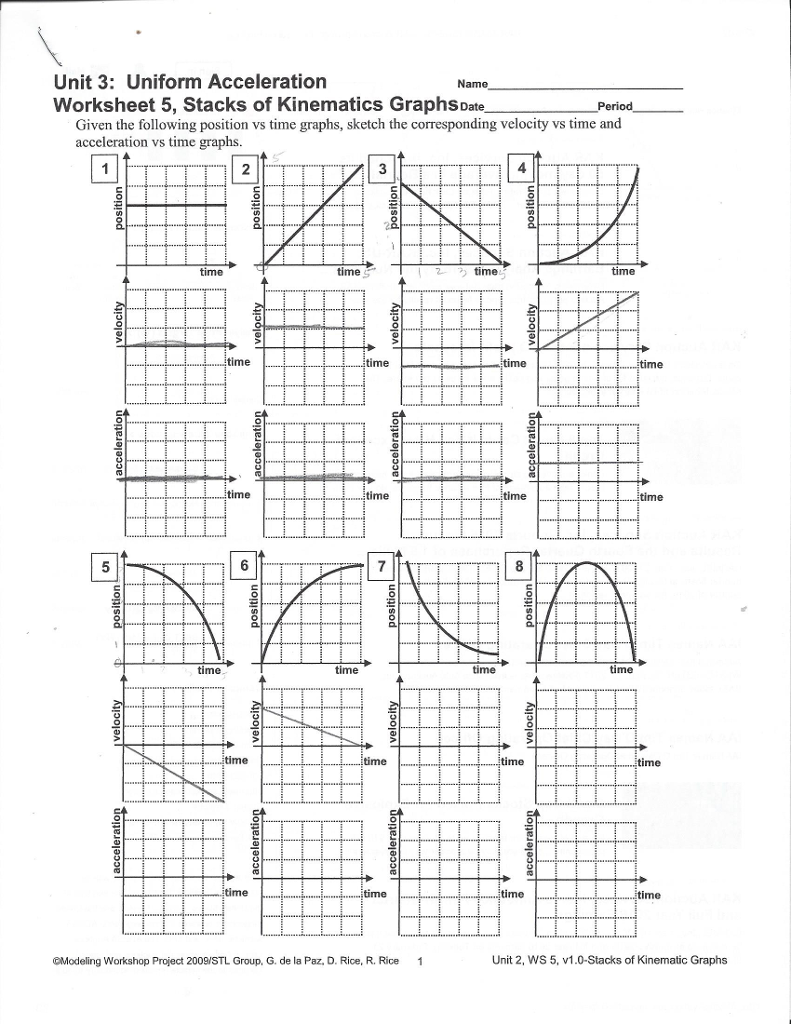Username: Password: Remember?×

 Divinity DarkRP Players 2/128

 Learn calculus with jaysea
09-10-2019, 12:51 AM (This post was last modified: 09-10-2019 12:52 AM by Jaysea.)
Post: #1JayseaMr. One Way
Hello friends today you will learn calculus with Professor Jaysea.

Direct all questions and inquiries to my ass09-10-2019, 06:02 AM
Post: #2FryLord0612Ok. No09-10-2019, 07:16 AM
Post: #3NitromechSo this is how you base

Spoiler

09-10-2019, 07:47 PM (This post was last modified: 09-10-2019 07:48 PM by OoGaBooGaMan.)
Post: #4OoGaBooGaManWatermaloneThere's gon' be some stuff you gon' see that's gon' make it hard to smile in the future. But through whatever you see, through all the rain and the pain, you gotta keep your sense of humor. You gotta be able to smile through all this bullshit.
​​​​​​​09-11-2019, 07:15 PM
Post: #5Phillipclubs seals till 02/10/2020
This helps me hit my shots in fortnite +repThanks to Young Scooter for the Amazing Breathtaking Signatures!
09-11-2019, 09:18 PM
Post: #6Jared From Subwayi actually got a brain tumour trying to think with my 47 chromosomes (more than average!! :)) so no, i do not recommend

Ad Memoriam Derrick Eakes
You will be missed but not forgotten.
Jared From Subway (STEAM_0:1:85896379) has been banned for 0 Minutes by Legacy the Duck for the reason "not eating fresh"

Perms  [cost: \$22,825,000] because i'm unoriginal

Fists
Vape
Surrender
Cdm
Knife
Katana
Hl2 Pistol
Prop Increase
Ammo Increase
Crowbar
Extra Printer
Pocket Increase
TTS
Elites
Snowball
Camera
Stun Stick
Glock
357
Frame Increase
Storage Increase
Camera Increase
Deagle
Dab
USP
Oil Increase
Fidget Spinner
09-11-2019, 09:38 PM
Post: #7KindredKeyboard Warrior
??? I required calc 1 - 3 to get my associates  jaysea please hnelp!

I look att hese pictuire and have irrational angry thjoughtsr
09-15-2019, 06:09 PM (This post was last modified: 09-15-2019 06:15 PM by Wolfgang Amadeus Mozart.)
Post: #8Wolfgang Amadeus MozartSuper Sexministrator
maths is a subject invented by the whiteys to oppress the black community
09-15-2019, 08:23 PM
Post: #9FryLord0612maths is a subject invented by the blacks to oppress the white community09-15-2019, 11:04 PM
Post: #10LiljayTurtle Boii
Without the incorrect equation to jaysea's problem with flying to subway

The remainder is like 2.309-16-2019, 12:42 AM (This post was last modified: 09-16-2019 01:00 AM by HarryFromSL.)
Post: #11HarryFromSLIn physics, velocity is how an object's position changes over time.
Physics students typically first learn this as the slope of a position-time graph.
However, the slope of a function is the same as the derivative of that function.
Thus, you can find an object's velocity through differentiation, using calculus.Consider the position-time graph above. It maps the function f(s) = 10s .
The object's coordinates started at (0,0) and moved to (50,5). We can divide the change in y over the change in x to find the slope of this position-time graph, and thus, the object's velocity. The velocity is 10 m/s.
Let's try getting the same answer using differentiation.Above is the power rule of derivatives: d/dx xn = n * xn-1
d/dx is notation for derivative. As stated before, the derivative of a function is the slope of the function.
d/dx 10^1s = 10 * (1 * s^(1-1))
d/dx 10^1s = 10 * (1*s^0)
d/dx 10^1s = 10 * (1*1)
d/dx 10^1s = 10 * 1
d/dx 10^1s = 10
The slope of the position-time graph is 10 m/s, whether you use the traditional slope method or derivatives. Differentiation was not very useful here, but it is in many scenarios.
We would write this as f'(m) = 10 .

Now, consider that acceleration is how an object's velocity changes over time. This means that acceleration is the slope of a velocity-time graph. We already learned that velocity is the slope of a position-time graph. Therefore, acceleration is the slope of the slope of a position-time graph.
Since the slope of a function is also the derivative of a function, this also means that acceleration is the derivative of a velocity-time graph, velocity is the derivative of a position-time graph, and acceleration is the derivative of the derivative of a position-time graph.
We already learned that the original function, which showed position over time, is named f(m) , and its derivative is f'(m) , which graphs velocity over time. The derivative of the derivative of f(m) , or the second derivative of f(m) , is then called f''(m) , which graphs acceleration over time.
I know this is a lot to take in. Hopefully these graphs may make sense to you.Back to finding f''(m).
If f'(m) = 10 , then f''(m) = d/dx 10 .
Here's a tip -- the derivative of any constant (for example, 10) is always 0. Why?
The derivative of a function is the slope of a function. The slope of a constant is always 0.Above is the graph for y=10 . Notice how the line is horizontal, and does not move up or down. The slope is 0, so the derivative is 0.
f''(m) = d/dx 10 = 0

What on earth did we just do?
We found the acceleration of the object! (that is, the slope of the velocity-time graph).
The object has no acceleration. Its position changed linearly over time, so its velocity was constant, and therefore it had no acceleration. You can see this phenomenon on the kinematic curves sheet.
What's really cool about the way we just did though, is that we didn't use some boring old slope formula. We used calculus, so we can apply this to some really crazy stuff later.
Show this to your friends when they say calculus has no real-world application.
o7

Oh and by the way, this relates to what Jaysea was saying about derivatives too. If you have some really funky curve going on you can slap a tangent line somewhere, and the slope of that is the derivative of the original curvy function. Super helpful.09-16-2019, 12:47 AM
Post: #12JayseaMr. One Way
Good job